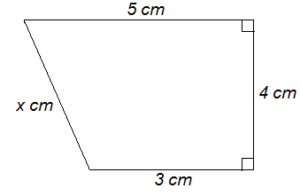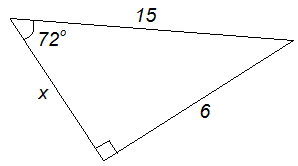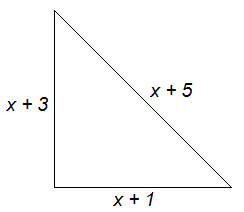Sat. Sep 30th, 2023

# JEXPO 2019 Mathematics Answer Key

Jun 11, 2019

### JEXPO 2019 Mathematics Answer Key

Note : JEXPO 2019 (WB Polytechnic) was of 100 MCQs among which 50 MCQs on Mathematics, 25 MCQs on Physics and 25 MCQs on Chemistry. Total of 100 MCQs was given in the puzzling form. I have enlisted here only 50 MCQs related to Mathematics.

[Solved] JEXPO 2019 – Physics and Chemistry Answer Key

Q. 1)The length of   equals

(A) 6 cm

(B)  cm

(C)

(D)

Q.2) Evaluate :

(A) 1

(B) 10

(C) 100

(D) 1000

Q.3)  where  and . Then values of  and   are

(A)

(B)

(C)

(D)

Q.4) The ages of Ram and Shyam differ by 16 years. 6 years ago, Shyam’s age was thrice as that of Ram’s. Find their present ages.

(A) 14 years, 30 years.

(B) 12 years, 28 years.

(C) 16 years, 34 years.

(D) 18 years, 38 years.

Q.5)  The simplification of    yields the result

(A)

(B)

(C)

(D)

Q.6) If the mean of 5 observations  and  is , find the value of   .

(A) 35

(B) 17

(C) 7

(D) 25

Q.7) If 12 men or 16 women can do a work in 172 days, how long will 21 men and 15 women to do the same work ?

(A) 64 days

(B) 60 days

(C) 86 days

(D) 75 days

Q.8) If  , then the value of    is :

(A)

(B)

(C)

(D)

Q.9) A chord of length 30 cm is at a distance of 8 cm from the centre of circle. The radius of circle is

(A) 16 cm

(B) 17 cm

(C) 12 cm

(D) 8 cm

Q.10) The correct expression to find    is :(A)

(B)

(C)

(D)

[Solved] JEXPO 2019 – Physics and Chemistry Answer Key

Q.11) The ratio of cost price and selling price is 4 : 5. The profit percent is

(A) 10%

(B) 20%

(C) 25%

(D) 30%

Q.12) Find the area of the square field if a train 800 metres long passes the field with a speed of 120 kmph in one minute.

(A) 1.44 sq.km

(B) 4 sq.km

(C) 2 sq.km

(D) 2.64 sq.km

Q.13)

(A) 3

(B) 4

(C) 6

(D) greater than 6

Q.14) In the quadratic equation  ,  if the sum of roots is 1.1, what is the product of the two roots ?

(A) 4

(B) 4.2

(C) 8

(D) None of the above.

Q.15) Sum of all natural numbers lying between 250 and 1000 which are exactly divisible by 3 is

(A) 157365

(B) 153657

(C) 156375

(D) 155637

Q.16) The principle that will yield Rs. 60 as simple interest at 6% per annum in 5 years is

(A) Rs. 175

(B) Rs. 350

(C) Rs. 200

(D) Rs. 150

Q.17) Three of the four expressions (A), (B), (C) and (D) given below are exactly equal. Which of the expression is not equal to the other three expressions ?

(A)

(B)

(C)

(D)

Q.18) Factorise :

(A) (a + 3)(a − 2)

(B) 3(a − 3)(a + 2)

(C) 3(a − 3)(a − 2)

(D) 3(a + 3)(a − 2)

Q.19) The value of    is :(A) 4

(B) 5

(C) 6

(D) 3

Q.20)

(A)

(B)

(C)

(D)

[Solved] JEXPO 2019 – Physics and Chemistry Answer Key

Q.21) If  then  is

(A)

(B)

(C)

(D)

Q.22) If  , then what is the value of

(A)

(B) 1.2934

(C) 1.29

(D) 1.295

Q.23) For a candidate to clear an examination, he/she must score 55% marks. If he/she gets 120 and fails by 78 marks, the total marks for the examination is

(A) 300

(B) 360

(C) 400

(D) 320

Q.24) The complement of    is

(A)

(B)

(C)

(D)

Q.25) In , then

(A)

(B)

(C)

(D)

Q.26) Find the point of intersection of graphs of

(A) (1, 1)(1, 4)

(B) (2, 4)(1, 1)

(C) (1, − 1)(2, 4)

(D) (− 2, 4)(1, 1)

Q.27) What is the value of  in

(A) 6

(B) 5

(C)

(D) − 11

Q.28) Two supplementary angles are in the ratio 3 : 2. The smaller angle measures

(A)

(B)

(C)

(D)

Q.29) The sum of digits of a two-digit number is 8. If the digits are reversed the number is increased by 54. Find the number.

(A) 17

(B) 19

(C) 21

(D) 23

Q.30) The 9th term of an A.P. is 449 and 449th term is 9. The term which is equal to zero is

(A) 508th

(B) 502th

(C) 501th

(D) None of the above

[Solved] JEXPO 2019 – Physics and Chemistry Answer Key

Q.31) of a number is 60. The number is

(A) 300

(B) 400

(C) 450

(D) 1200

Q.32) What is the least number to be added to 920 to make it a perfect square ?

(A) 41

(B) 31

(C) 39

(D) 49

Q.33) If   , then T is equal to

(A)

(B)

(C)

(D)

Q.34) A man cycles at a rate of 4 kmph. He cycles for 45 minutes and then takes a 15 minutes break. Determine the total time taken to cover 12 km by him.

(A) 4 hours 35 minutes

(B) 4 hours

(C) 3 hours

(D) 3 hours 45 minutes

Q.35) Three coins are tossed. What is the probability of getting at most two tails ?

(A)

(B)

(C)

(D)

Q.36) Find the ratio in which the line joining the points (6, 4) and (1, − 7) is divided by x-axis.

(A) 1 : 3

(B) 2 : 7

(C) 4 : 7

(D) 6 : 7

Q.37) What will come in place of  ‘a’  in the following expression ?

a − 796.21 + 498.05 = 215.50 − 425.01

(A) 71.81

(B) 81.71

(C) 88.65

(D) None of the above.

Q.38) If  , then is equal to

(A) 0

(B) 1

(C) sum of their roots

(D) product of roots

Q.39) Given :  . The greatest and least of them respectively

(A)

(B)

(C)

(D)

Q.40) In a poultry farm having hens and pigs. Rohan can see 84 heads and 282 legs. How many hens are there ?

(A) 54

(B) 30

(C) 27

(D) 57

[Solved] JEXPO 2019 – Physics and Chemistry Answer Key

Q.41) Length of a rectangle is 12 cm more than its breadth and it has a perimeter of 200 cm. What will be the diameter of a circle whose area matches the area of this rectangle ?

(A) 44 cm

(B) 56 cm

(C) 28 cm

(D) 45 cm

Q.42) If one is added to the numerator of the fraction it becomes 1. If one is added to the denominator of the fraction it becomes . The fraction is

(A)

(B)

(C)

(D)

Q.43)  is expressed in the form . The values of a and b are

(A) a = 3, b = 8

(B) a = − 3, b = − 10

(C) a = − 3, b = − 8

(D) a = − 3, b = 10

Q.44) Two numbers are less than a third number by 30% and 37% respectively. How much percent is the second number less than the first ?

(A) 7%

(B) 10%

(C) 4%

(D) 3%

Q.45) Shyam has a solid metal ball with diameter 6 cm. He melts it and uses the material for making a solid cylinder. If the diameter of the cylinder is same as the ball, what would its height be ?

(A) 4 cm

(B) 4.5 cm

(C) 6 cm

(D) 8 cm

Q.46) How many numbers between 100 and 600 are divisible by 2, 3 and 7 together ?

(A) 11

(B) 12

(C) 14

(D) cannot be determined

Q.47) Two numbers are in the ratio 5 : 6. If their H.C.F. is 4, then their L.C.M. will be

(A) 90

(B) 96

(C) 120

(D) 150

Q.48) Find the least values of x and y so that the number is divisible by 88

(A) 8, 2

(B) 7, 3

(C) 9, 4

(D) 6, 5

Q.49) The coordinates of the point of intersection of two graphs could only be(A) (− 1, 2)

(B) (− 1, − 2)

(C) (1, 2)

(D) (2, − 1)

Q.50) Minimum possible interior angle in a regular polygon is

(A)

(B)

(C)

(D)

[Solved] JEXPO 2019 – Physics and Chemistry Answer Key

Thank You

#### Navigating JENPAUH 2016: Your Gateway to a Bright Medical Career

error: Content is protected !!